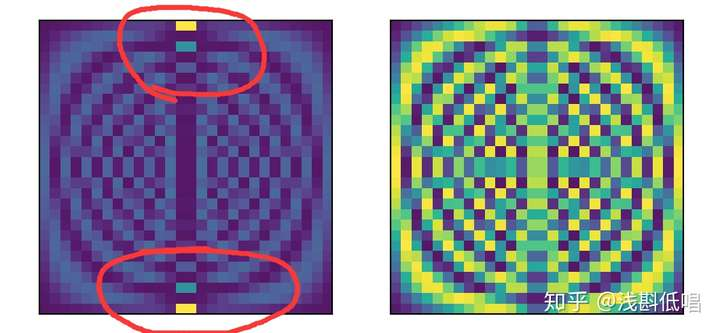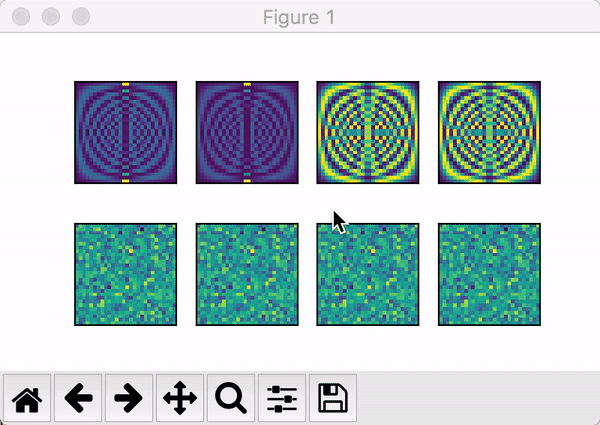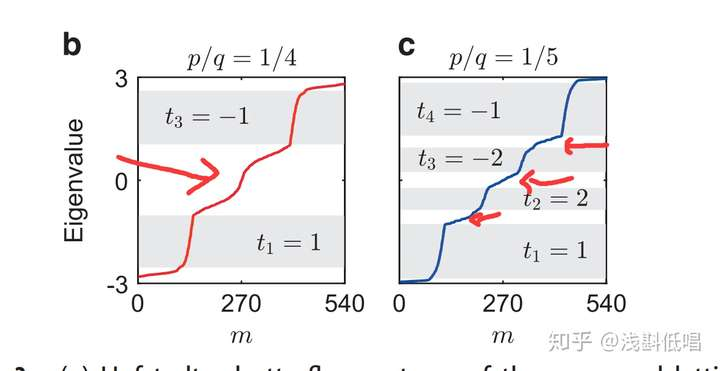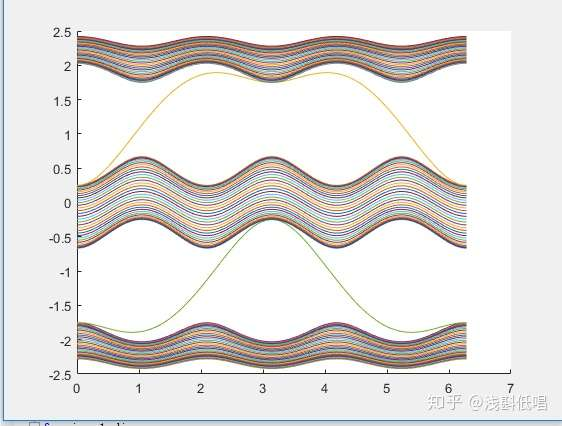## Detect topological phase transition in SSH model by unsupervised learning

This is an English version (and a slightly advanced one)  of my post in ZhiHu website.

The most fascinating and promising technique in CS field is artificial intelligence; while the most interesting concepts in modern physics and condensed matter field are those phase transitions beyond Landau’s theory.

Recently, the machine learning techniques have been used to detect the phase boundary, such as, . In his article, using the PCA(Principai Component Analysis, a method in unsupervised learning. )  to analysis the ES(entanglement spectrum, a quantum information quantity of many body ground state) of the Kitaev model, authors claim that this method is helpful in finding the topological phase transition. However, ES is a relatively advanced concept and the success of PCA may be based on the success of information compressing of ES. I would love to point out that, in finite size sheet, due to the gapless excitations on the edge whose energy is in the gap of the infinite size sheet, the topological phase transition can be easily confirmed only by learning the spectrum or wave function. We do not need to process any data by ES analysis.The figure is from the paper.

The idea comes from one of my thought: Why physics can be learned by a machine?  Actually, stuff in physics is more convenient for a machine to learn. An intuitive way to understand this fact is that, for local Hamiltonian of a quantum system, there exists so-called domain wall; and in the time domain, we know that. Therefor, no matter your system is N-dimensional statistical local mechanics or N+1- dimensional quantum dynamics, the conventional RNN, CNN is very suitable. Because in basic machine learning course we know that this network is very suitable for learning short-range correlation.

Here I use another famous method, autoencoder, to demonstrate how machine learning technique helps to detect phase transition. To simplify our discussion, by default we suppose all of the readers are very familiar to autoencoder. We mainly focus on the SSH model which also is very familiar to readers (by default).

The Hamiltonian of SSH model is rather simple, i.e,The topological phase is when. Because the system is quadric(thus there is no interaction), serveral commands can generate the Hamiltonian of the system:

diag1 = np.array(
[1 + delta_t*(-1)**(i) for i in range(2*L-1)],
dtype = complex,
)
H = np.diag(diag1,-1) +np.diag(diag1,1)

Then, we can diagonalise the system very simply:

w,v = blas.eigh(H)
N=np.real(np.conj(v)*v)

Then, the n-th row of N corresponds to the n-th eigenenergy in w. Plotting it, we have:See the red circle, which is our target(the edge state of SSH model).

By adding random on-site disorder, we can generate many data from this process. Now we can define a network. The package I used is pyTorch:

class AutoEncoder(nn.Module):
def __init__(self):
super(AutoEncoder, self).__init__()

self.encoder = nn.Sequential(
nn.Linear(4*L*L, 128),
nn.ReLU(),
nn.Linear(128, 64),
nn.ReLU(),
nn.Linear(64, 2),
)
self.decoder = nn.Sequential(
nn.Linear(2, 64),
nn.ReLU(),
nn.Linear(64, 128),
nn.ReLU(),
nn.Linear(128, 4*L*L),
)

def forward(self, x):
encoded = self.encoder(x)
decoded = self.decoder(encoded)
return encoded, decoded

I do not want to say more about this autoencoder beacuse it is so fundamental.

We use Adam to optimize and LSE as our loss function. One thing I want to note is that, our wave function is purely real! I collect 8000+ data and patch them to 32 groups, shuffle it into the training pour, we can have this feature clustering very quickly:Where the label 1 denote topological phase and 0 denotes the normal phase. The convergent time is so short and for your convenience I delibarately slow down the process to obtain:We see, learning from wave function is very effectient and trvial. To demonstrate my idea of learning from spectrum, we have the Hofstadter model, (see Aidelsburg’s phd thesis). We can plot the spectrum for a finite size square lattice when there exist four or five bands:We will see that the number of eigenvalues in gapisof the total number of eigenvalues, where l is the perimeter of the sheet.Any unsupervised learning method can learn the spectrum easily. Thus, we can detect the topological properties even easier.

## Note on 1D-Bosonization Technique

Physics does not depend on the representation, but your life surely does. In this short note, we review the basic bosonization method for studying the interacting 1D system [and then we review the rigorous developments and techniques. Several applications will Read more…

## Quantum Anomalous Hall Effect in Kagome Lattice: Topological Band Study

The main result is still being calculated. See band structure in my notebook: http://nbviewer.jupyter.org/github/caidish/my_nb/blob/master/Kagome_lattice.ipynb The edge mode is also interesting. Chern number is also calculated. http://nbviewer.jupyter.org/github/caidish/my_nb/blob/master/Kagome_lattice_edge_mode.ipynb